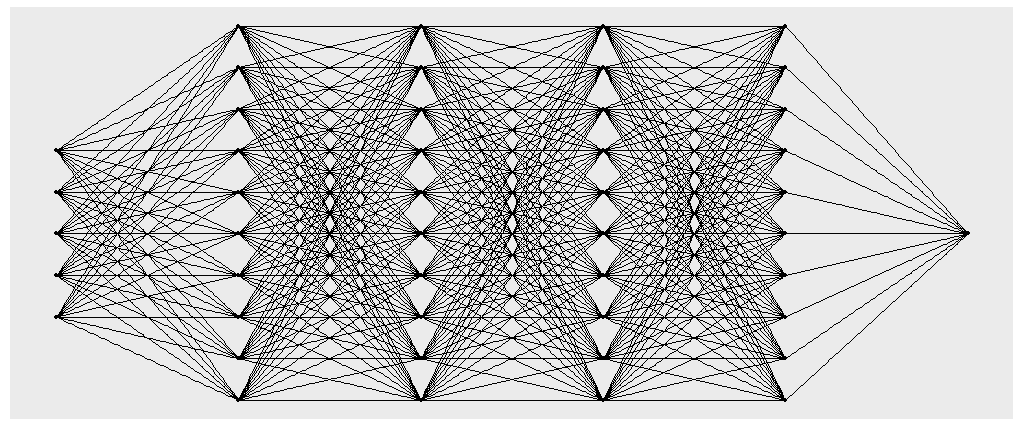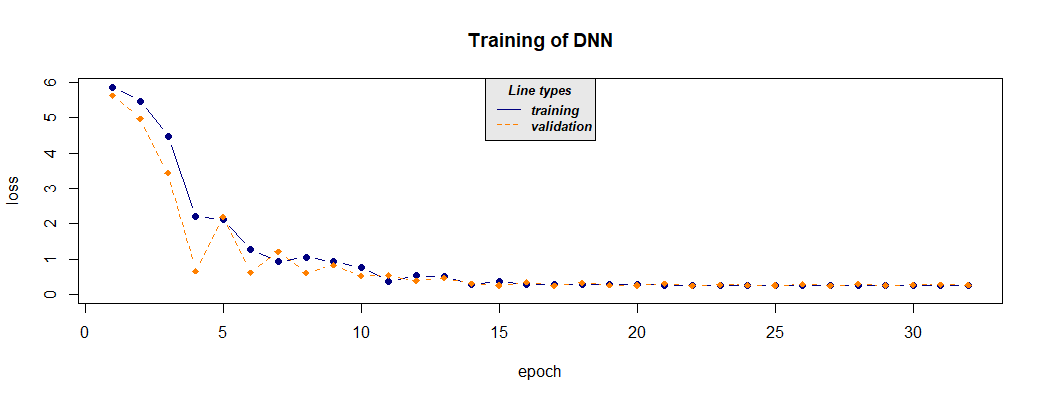# cito

## Abstract

‘cito’ allows you to build and train Neural Networks with the standard R syntax. The whole process of generating and training a deep neural network can be done with command. Afterwards all generic R methods can be used on the model, which makes comparisons way easier. It is based on the ‘torch’ machine learning framework which for R. Since it is native to R, no Python installation or API is needed for this package.

## Setup

### Installing torch

Before using cito for the first time, make sure that the current version of ‘torch’ is installed running.

if(!require(torch)) install.packages("torch")
library(torch)
if(!torch_is_installed()) install_torch()

library (cito)

### Data

As an example we will work with the irirs dataset. Which consists of three species and four descriptive variables.

data <- datasets::iris
#>   Sepal.Length Sepal.Width Petal.Length Petal.Width Species
#> 1          5.1         3.5          1.4         0.2  setosa
#> 2          4.9         3.0          1.4         0.2  setosa
#> 3          4.7         3.2          1.3         0.2  setosa
#> 4          4.6         3.1          1.5         0.2  setosa
#> 5          5.0         3.6          1.4         0.2  setosa
#> 6          5.4         3.9          1.7         0.4  setosa

#scale dataset
data <- data.frame(scale(data[,-5]),Species = data[,5])

## Introduction to models and model structures

cito allows you to use the formula syntax to build multi layer perceptron networks. Here we build a network with 4 Hidden layers, each with 10 perceptrons. You do not need to adjust factors or include interactions as data columns manually this can be done through the formula syntax.

While this training is done on the cpu, all models can be trained on the gpu with device = “cuda”.

library(cito)
nn.fit <- dnn(Sepal.Length~. , data = data, hidden = c(10,10,10,10), epochs = 12, device = "cpu")
#> Loss at epoch 1: 0.906718, lr: 0.01000
#> Loss at epoch 2: 0.863654, lr: 0.01000
#> Loss at epoch 3: 0.843066, lr: 0.01000
#> Loss at epoch 4: 0.825574, lr: 0.01000
#>
#> ....
#>
#> Loss at epoch 11: 0.408130, lr: 0.01000
#> Loss at epoch 12: 0.403822, lr: 0.01000

You can plot the networks structure to give you an overview of the created object. Be aware that this may take some time for really big networks.

plot(nn.fit)Structure plot of the generate network

As standard all layers are fitted with relu as activation layer. $relu(x) = max (0,x)$ However, you can adjust the activation function of each layer individually to build exactly the network you want. In this case you have to provide a vector the same length as there are hidden layers. The activation function for the output layer (also called “link” function) is chosen automatically and does not have to be provided.

#selu as activation function for all layers:
nn.fit <- dnn(Sepal.Length~., data = data, hidden = c(10,10,10,10), activation= "selu")
#layer specific activation functions:
nn.fit <- dnn(Sepal.Length~., data = data,
hidden = c(10,10,10,10), activation= c("relu","selu","tanh","sigmoid"))

### Adding a validation set to the training process

In order to see where your model might start overfitting the addition of a validation set can be useful. In dnn() you can put activation = 0.x and the defined percentage will be not used for training and only for validation after each epoch.

#20% of data set is used as validation set
nn.fit <- dnn(Sepal.Length~., data = data, epochs = 32,
loss= "mae", hidden = c(10,10,10,10), validation = 0.2)
#> Loss at epoch 1: training: 5.868, validation: 5.621, lr: 0.01000
#> Loss at epoch 2: training: 5.464, validation: 4.970, lr: 0.01000
#> Loss at epoch 3: training: 4.471, validation: 3.430, lr: 0.01000
#> Loss at epoch 4: training: 2.220, validation: 0.665, lr: 0.01000
#>
#> ...
#>
#>
#> Loss at epoch 31: training: 0.267, validation: 0.277, lr: 0.01000
#> Loss at epoch 32: training: 0.265, validation: 0.275, lr: 0.01000Training loss

The validation loss allows us to choose the model which has the minimum validation loss as out optimal one. By setting nn.fit$use_model_epoch to the model with the least validation loss, from now on always this model will be used for all following functions nn.fit$use_model_epoch <- which.min(nn.fit$losses$valid_l)

### Interpreting model output

The standard S3 functions can be used to interpret the model:

#utilize model on new data
predict(nn.fit,data[1:3,])
#>          [,1]
#> [1,] 5.046695
#> [2,] 4.694821
#> [3,] 4.788142
#returns weights of neural network
coef(nn.fit)
#> []
#> []$0.weight #> [,1] [,2] [,3] [,4] [,5] [,6] #> [1,] 0.21469544 0.17144544 0.06233330 0.05737647 -0.56643492 0.30539653 #> [2,] 0.02309913 0.32601142 -0.04106455 -0.05251846 0.06156364 -0.16903549 #> [3,] 0.02779424 -0.39305094 0.22446594 -0.11260942 0.40277928 -0.14661779 #> [4,] -0.17715086 -0.34669805 0.41711944 -0.07970788 0.28087401 -0.32001352 #> [5,] 0.10428729 0.46002910 0.12490098 -0.25849682 -0.49987957 -0.19863304 #> [6,] 0.08653354 0.02208819 -0.18835779 -0.18991815 -0.19675359 -0.37495106 #> [7,] 0.28858119 0.02029459 -0.40138969 -0.39148667 -0.29556298 0.17978610 #> [8,] 0.34569272 -0.04052169 0.76198137 0.31320053 -0.06051779 0.34071702 #> [9,] 0.34511277 -0.42506409 -0.50092584 -0.22993636 0.05683114 0.38136607 #> [10,] -0.13597916 0.25648212 -0.08427665 -0.46611786 0.14236088 0.04671739 #> #> ... #> #> []$8.bias
#>  0.2862495

Feature Importance based on Fisher, Rudin, and Dominici (2018)

# Calculate and return feature importance
summary(nn.fit)
#> Deep Neural Network Model summary
#> Feature Importance:
#>      variable importance
#> 1  Sepal.Width   3.373757
#> 2 Petal.Length   3.090394
#> 3  Petal.Width   2.992742
#> 4      Species   3.278064

## Training parameter for the Neural Network

Training a neural network can be an art of itself. Fitting a neural network usually takes some trial and error.

### Regularization

Neural Networks tend to overfit to your dataset. Therefore it is best to use some kind of regularization. In dnn() you can use elastic net regularization and dropout layers.

#### L1/L2 Regularization

The L1/L2 loss is controlled by alpha and lambda, where the loss is simply added to the general loss of the network.

$L1/L2 loss = \lambda * [ (1 - \alpha) * |weights| + \alpha |weights|^2 ]$

The optimizer will try to also minimize the equation above which can lead to a nice generalization and avoids over fitting.

If a single alpha value is provided each layer w ill get regularized the same. However, you can regularize each layer individually by providing a vector of alpha values the same length as there are hidden layers + 1, since the input layer also has weights that are relevant. Since FALSE gets converted to 0 in an numeric vector you have to enter NA if no penalty should be added on a specific layer.

#elastic net penalty in all layers:
nn.fit <- dnn(Species~., data = data, hidden = c(10,10,10,10), alpha = 0.5, lambda = 0.01)
#L1 generalization in the first layer no penalty on the other layers:
nn.fit <- dnn(Species~., data = data, hidden = c(10,10,10,10),
alpha = c(0,NA,NA,NA,NA), lambda = 0.01)

#### Dropout Regularization

Dropouts are another way to regularize your model. During training in every epoch a perceptrons has a chance to get left out. You can control this percentage with the dropout parameter.

#dropout of 35% on all layers:
nn.fit <- dnn(Species~., data = data, hidden = c(10,10,10,10), dropout = 0.35)
#dropout of 35% only on last 2 layers:
nn.fit <- dnn(Species~., data = data,
hidden = c(10,10,10,10), dropout = c(0, 0, 0.35, 0.35))

### Learning rate

The lr parameter controls the so called learning rate. This parameter defines the step size the optimizer takes during training at each epoch. If set too high the optimizer might not find a good optimum because you shoot across certain valleys. If set too low the training process takes too long to be viable.

### Learning rate scheduler

Learning rate scheduler are allow you to start with a high learning rate and decrease it during the training process. You can choose between different types of schedulers. Namely, lambda, multiplicative, one_cycle and step.

The function config_lr_scheduler() helps you setup such a scheduler. See ?config_lr_scheduler() for more information

# Step Learning rate scheduler that reduces learning rate every 16 steps by a factor of 0.5
scheduler <- config_lr_scheduler(type = "step",
step_size = 16,
0.5)

nn.fit <- dnn(Sepal.Length~., data = data,lr = 0.01, lr_scheduler= scheduler)

### Optimizer

Optimizer are responsible for calculating the direction when updating weights in the training process. The optimizer always tries to minimize the loss function. As standard the adam optimizer will be used.

However, you can customize your optimizer with config_optimizer() and use other types of optimizer and adjust the hyperparameter of you optimizer. See ?config_optimizer() for more information.


# adam optimizer with learning rate 0.002 with slightly changed betas to 0.95, 0.999 and eps to 1.5e-08
opt <- config_optimizer(
betas = c(0.95, 0.999),
eps = 1.5e-08)

nn.fit <- dnn(Species~., data = data, optimizer = opt, lr=0.002)

### Loss functions

Loss function evaluate the current residuals and measure how good the network performs. Of course the standard Loss functions like mean absolute error, real mean squared error and cross-entropy are implemented , you can also fit to certain probability distributions.

# Real Mean squared error
nn.fit <- dnn(Sepal.Length~. data = data, loss = "rmse")

# Fit to a normal distribution, you can also define the parameters of the distribution
nn.fit <- dnn(Sepal.Length~. data = data, loss = stats::gaussian()) 

### Early Stopping

Adding early stopping criteria helps you save time by stopping the training process early, if the validation loss of the current epoch is bigger than the validation loss n epochs early. The n can be defined by the early_stopping argument. It is required to set validation > 0.

# Stops training if validation loss at current epoch is bigger than that 15 epochs earlier
nn.fit <- dnn(Sepal.Length~., data = data, epochs = 1000,
validation = 0.2, early_stopping = 15)

## Continue training process

In case you want to continue the training process of an existing model you need to use continue_training(). This function allows you to continue the training process where dnn() stopped.

# simple example, simply adding another 12 epochs to the training process
nn.fit <- continue_training(nn.fit, epochs = 12)

It also allows you to change any training parameters, for example the learning rate. Additional, you can define which epoch the training should continue from. So you can analyze the training process with analyze_training() and afterwards pick an epoch from which on the training should be continued from with other training parameters.


# picking the model with the smalles validation loss
# with changed parameters, in this case a smaller learning rate and a smaller batchsize
nn.fit <- continue_training(nn.fit,
continue_from = which.min(nn.fit$losses$valid_l),
epochs = 32,
changed_params = list(lr = 0.001, batchsize = 16))